# 011514 BELL WORK WRITE QUESTION AND ANSWER Do

• Slides: 33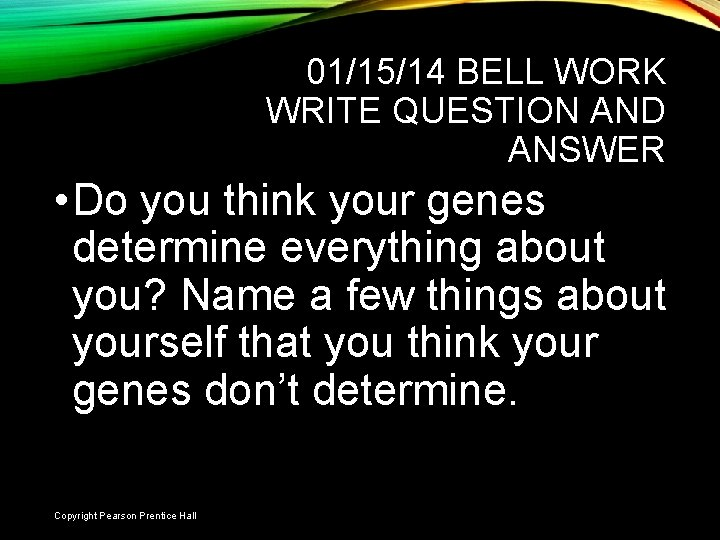01/15/14 BELL WORK WRITE QUESTION AND ANSWER • Do you think your genes determine everything about you? Name a few things about yourself that you think your genes don’t determine. Slide 1 of 21 Copyright Pearson Prentice Hall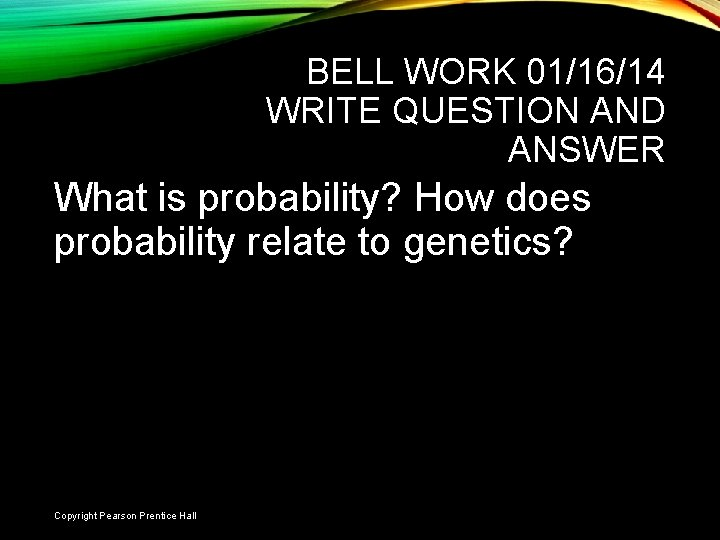• . BELL WORK 01/16/14 WRITE QUESTION AND ANSWER What is probability? How does probability relate to genetics? Slide 2 of 21 Copyright Pearson Prentice Hall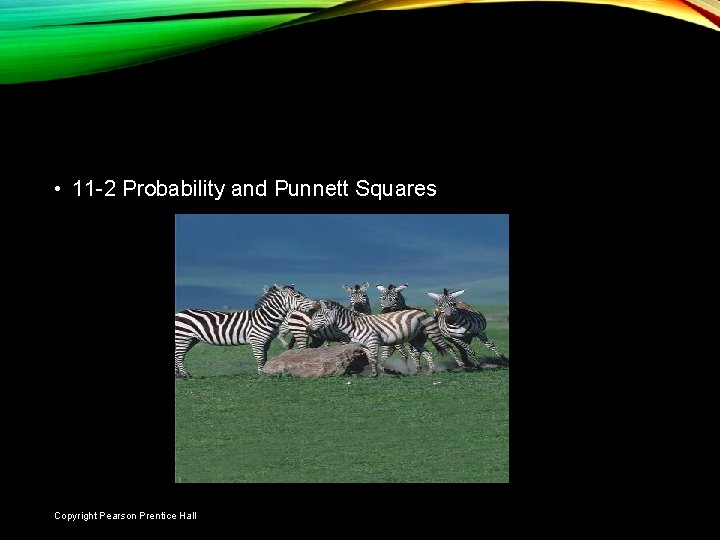11 -2 PROBABILITY AND PUNNETT SQUARES • 11 -2 Probability and Punnett Squares Slide 3 of 21 Copyright Pearson Prentice Hall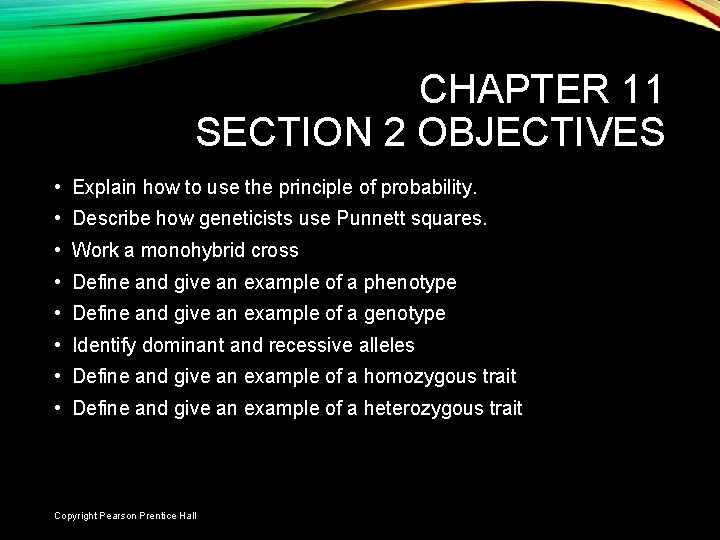CHAPTER 11 SECTION 2 OBJECTIVES • Explain how to use the principle of probability. • Describe how geneticists use Punnett squares. • Work a monohybrid cross • Define and give an example of a phenotype • Define and give an example of a genotype • Identify dominant and recessive alleles • Define and give an example of a homozygous trait • Define and give an example of a heterozygous trait Slide 4 of 21 Copyright Pearson Prentice Hall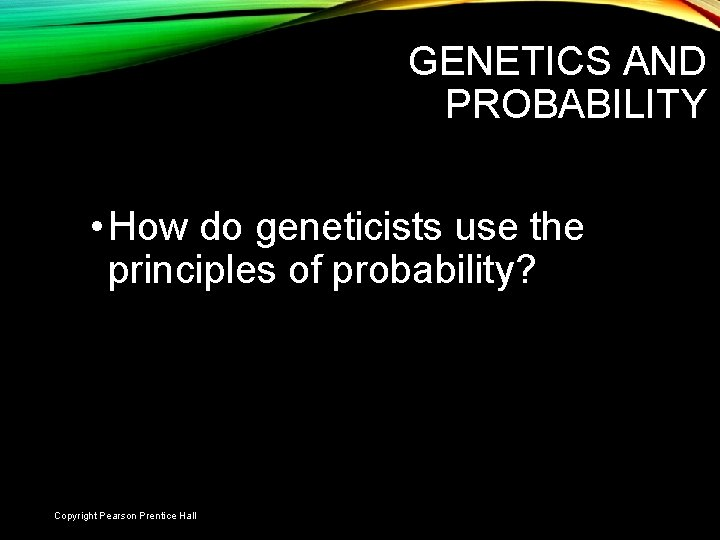GENETICS AND PROBABILITY • How do geneticists use the principles of probability? Slide 5 of 21 Copyright Pearson Prentice HallGENETICS AND PROBABILITY • Genetics and Probability • Probability - The likelihood that a particular event will occur. • The principles of probability can be used to predict the outcomes of genetic crosses. Slide 6 of 21 Copyright Pearson Prentice HallPUNNETT SQUARES • How do geneticists use Punnett squares? Slide 7 of 21 Copyright Pearson Prentice Hall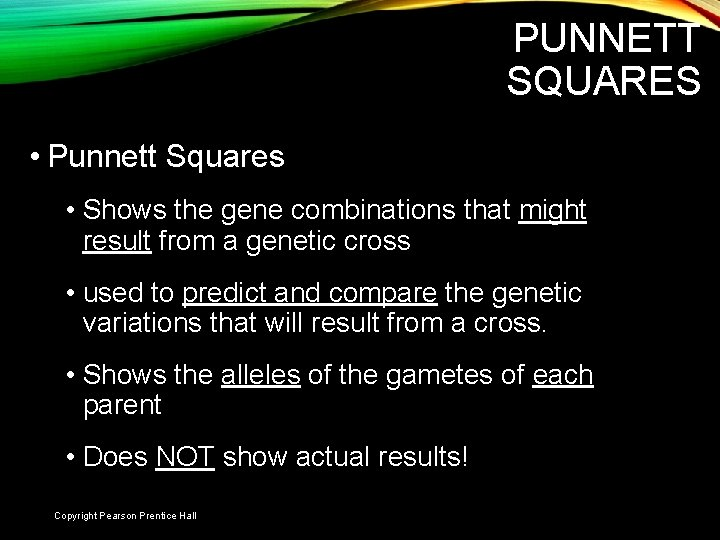PUNNETT SQUARES • Punnett Squares • Shows the gene combinations that might result from a genetic cross • used to predict and compare the genetic variations that will result from a cross. • Shows the alleles of the gametes of each parent • Does NOT show actual results! Slide 8 of 21 Copyright Pearson Prentice Hall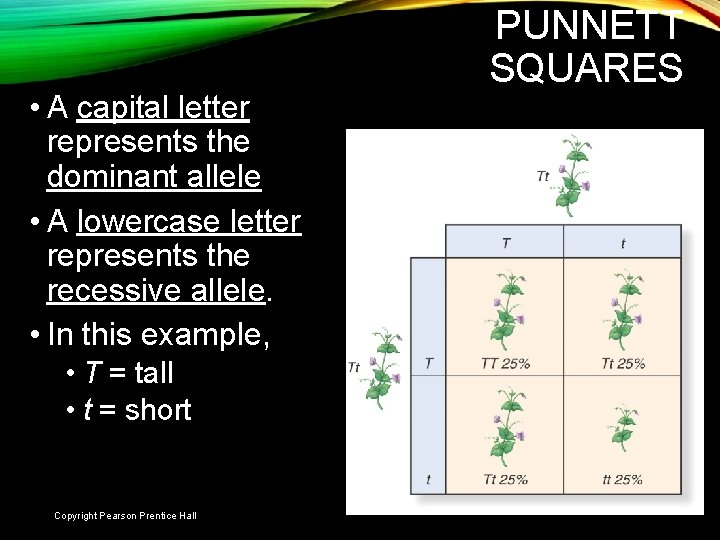• A capital letter represents the dominant allele • A lowercase letter represents the recessive allele. • In this example, PUNNETT SQUARES • T = tall • t = short Slide 9 of 21 Copyright Pearson Prentice Hall• Gametes are shown along the top and left side. PUNNETT SQUARES Slide 10 of 21 Copyright Pearson Prentice Hall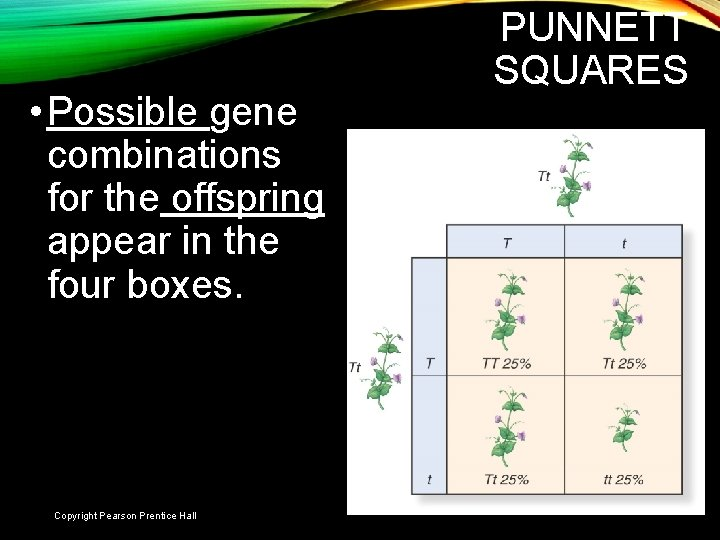• Possible gene combinations for the offspring appear in the four boxes. PUNNETT SQUARES Slide 11 of 21 Copyright Pearson Prentice Hall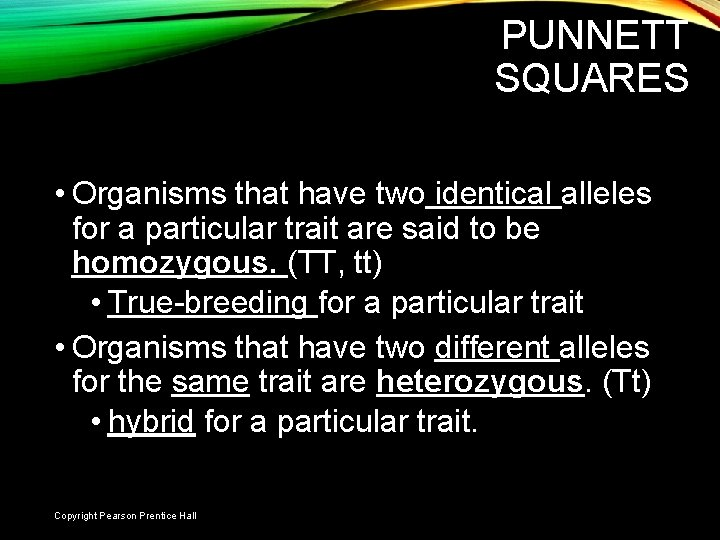PUNNETT SQUARES • Organisms that have two identical alleles for a particular trait are said to be homozygous. (TT, tt) • True-breeding for a particular trait • Organisms that have two different alleles for the same trait are heterozygous. (Tt) • hybrid for a particular trait. Slide 12 of 21 Copyright Pearson Prentice HallTABLE PRACTICE 1 • For each genotype, indicate whether it is heterozygous (HE) or homozygous (HO) dominant or recessive • AA Ii • Bb Jj • Cc kk • Dd Ll • Ee MM • ff nn • GG OO • HH Pp Copyright Pearson Prentice Hall Slide 13 of 21TABLE PRACTICE 1 • For each genotype, indicate whether it is heterozygous (HE) or homozygous (HO) dominant or recessive • AA – HO dominant Ii - HE • Bb - HE Jj - HE • Cc - HE kk – HO recessive • Dd - HE Ll - HE • Ee - HE MM – HO dominant • ff – HO recessive nn – HO recessive • GG – HO dominant OO – HO dominant • HH – HO dominant Copyright Pearson Prentice Hall Pp - HE Slide 14 of 21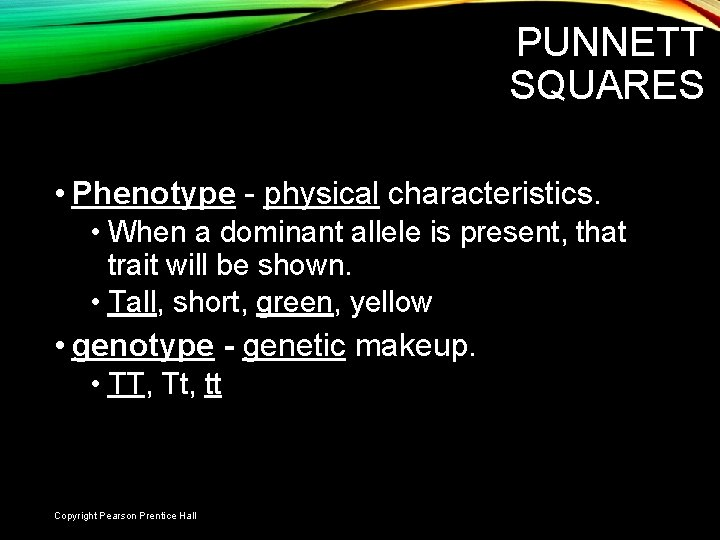PUNNETT SQUARES • Phenotype - physical characteristics. • When a dominant allele is present, that trait will be shown. • Tall, short, green, yellow • genotype - genetic makeup. • TT, Tt, tt Slide 15 of 21 Copyright Pearson Prentice Hall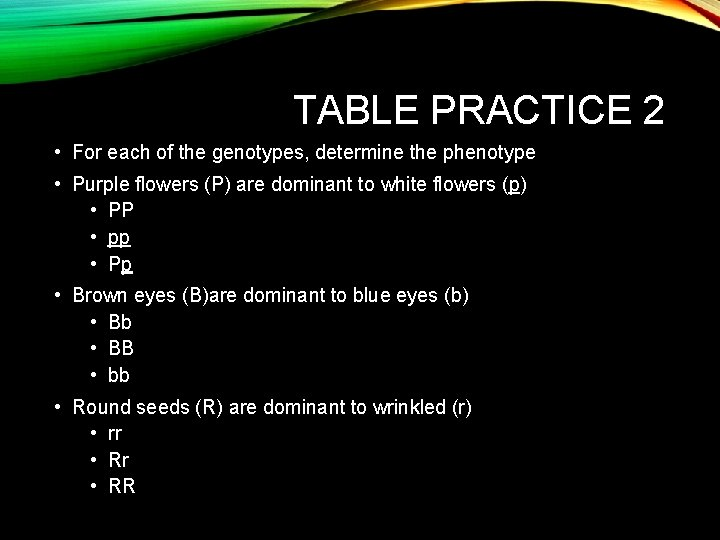TABLE PRACTICE 2 • For each of the genotypes, determine the phenotype • Purple flowers (P) are dominant to white flowers (p) • PP • pp • Pp • Brown eyes (B)are dominant to blue eyes (b) • Bb • BB • bb • Round seeds (R) are dominant to wrinkled (r) • rr • RR Slide 16 of 21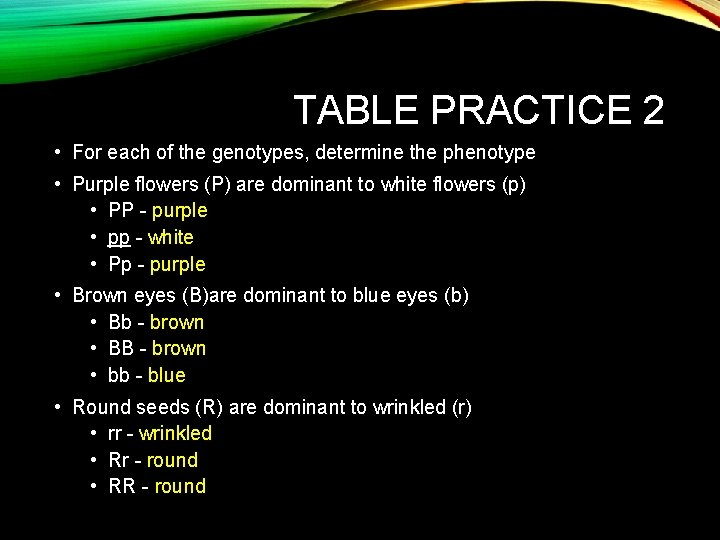TABLE PRACTICE 2 • For each of the genotypes, determine the phenotype • Purple flowers (P) are dominant to white flowers (p) • PP - purple • pp - white • Pp - purple • Brown eyes (B)are dominant to blue eyes (b) • Bb - brown • BB - brown • bb - blue • Round seeds (R) are dominant to wrinkled (r) • rr - wrinkled • Rr - round • RR - round Slide 17 of 21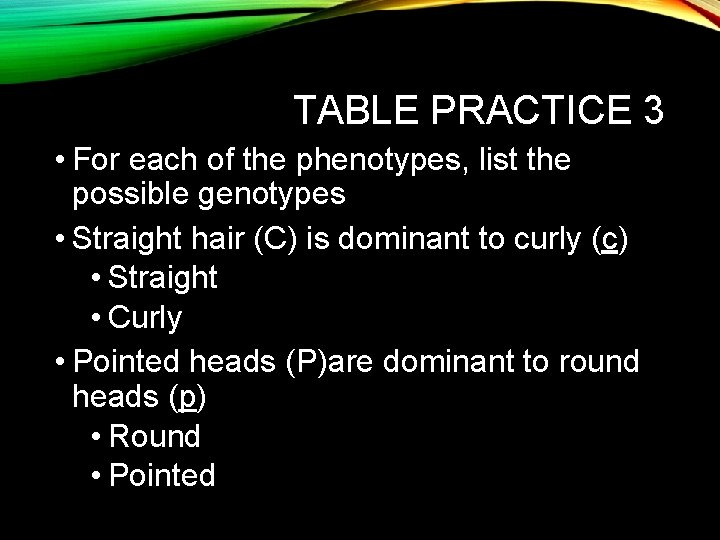TABLE PRACTICE 3 • For each of the phenotypes, list the possible genotypes • Straight hair (C) is dominant to curly (c) • Straight • Curly • Pointed heads (P)are dominant to round heads (p) • Round • Pointed Slide 18 of 21TABLE PRACTICE 3 • For each of the phenotypes, list the possible genotypes • Straight hair (C) is dominant to curly (c) • Straight – CC, Cc • Curly - cc • Pointed heads (P)are dominant to round heads (p) • Round - pp • Pointed – PP or Pp Slide 19 of 21PUNNETT SQUARES • An organism can have different genotypes (TT and Tt), but have the same phenotype (tall). Slide 20 of 21 Copyright Pearson Prentice Hall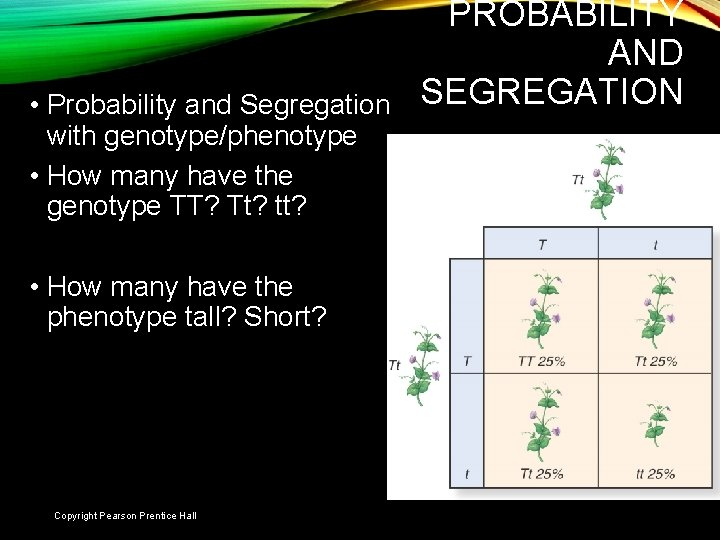• Probability and Segregation with genotype/phenotype • How many have the genotype TT? Tt? tt? PROBABILITY AND SEGREGATION • How many have the phenotype tall? Short? Slide 21 of 21 Copyright Pearson Prentice Hall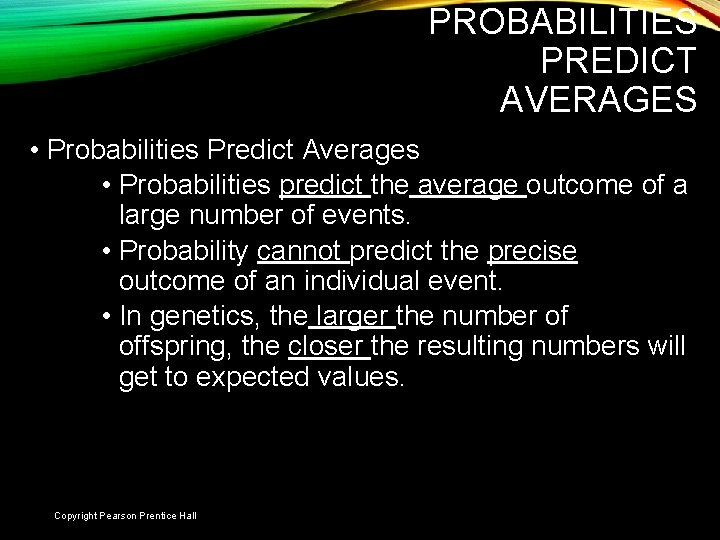PROBABILITIES PREDICT AVERAGES • Probabilities Predict Averages • Probabilities predict the average outcome of a large number of events. • Probability cannot predict the precise outcome of an individual event. • In genetics, the larger the number of offspring, the closer the resulting numbers will get to expected values. Slide 22 of 21 Copyright Pearson Prentice Hall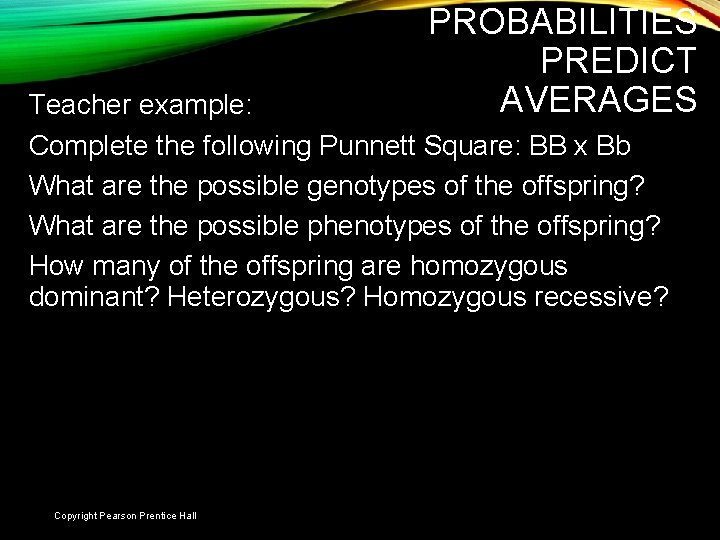PROBABILITIES PREDICT AVERAGES Teacher example: Complete the following Punnett Square: BB x Bb What are the possible genotypes of the offspring? What are the possible phenotypes of the offspring? How many of the offspring are homozygous dominant? Heterozygous? Homozygous recessive? Slide 23 of 21 Copyright Pearson Prentice Hall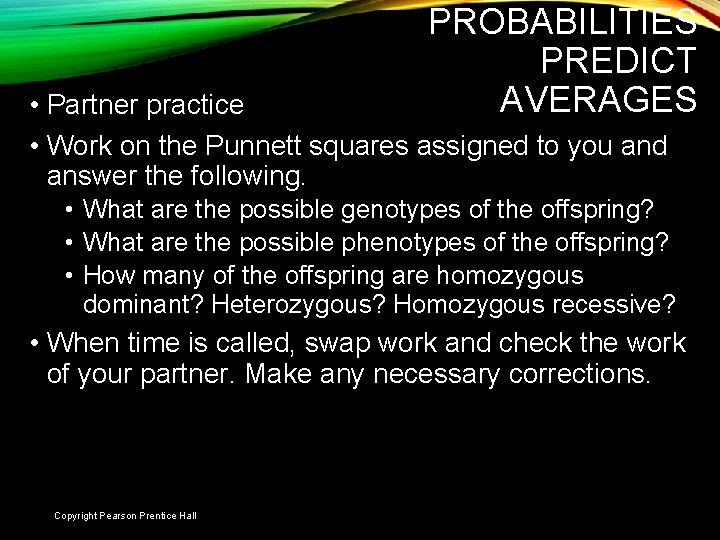PROBABILITIES PREDICT AVERAGES • Partner practice • Work on the Punnett squares assigned to you and answer the following. • What are the possible genotypes of the offspring? • What are the possible phenotypes of the offspring? • How many of the offspring are homozygous dominant? Heterozygous? Homozygous recessive? • When time is called, swap work and check the work of your partner. Make any necessary corrections. Slide 24 of 21 Copyright Pearson Prentice HallREMINDER • Chapter 11 Section 2 Workbook pages should be complete • Be ready for chapter 11 section 2 quiz Slide 25 of 21 Copyright Pearson Prentice Hall11 -2 Click to Launch: Continue to: - or - Slide 26 of 21 Copyright Pearson Prentice Hall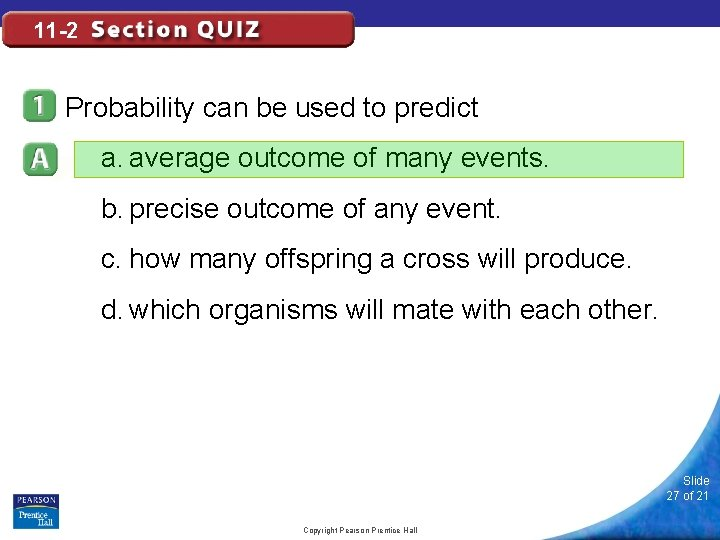11 -2 Probability can be used to predict a. average outcome of many events. b. precise outcome of any event. c. how many offspring a cross will produce. d. which organisms will mate with each other. Slide 27 of 21 Copyright Pearson Prentice Hall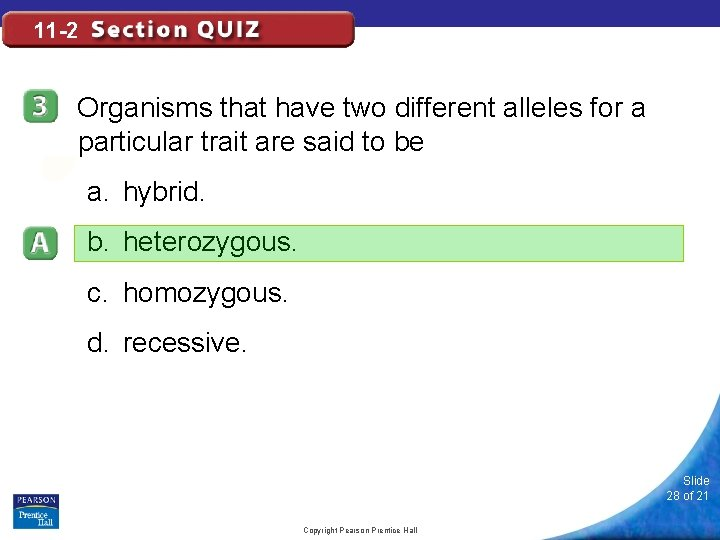11 -2 Organisms that have two different alleles for a particular trait are said to be a. hybrid. b. heterozygous. c. homozygous. d. recessive. Slide 28 of 21 Copyright Pearson Prentice Hall11 -2 Two F 1 plants that are homozygous for shortness are crossed. What percentage of the offspring will be tall? a. 100% b. 50% c. 0% d. 25% Slide 29 of 21 Copyright Pearson Prentice Hall11 -2 The Punnett square allows you to predict a. only the phenotypes of the offspring from a cross. b. only the genotypes of the offspring from a cross. c. both the genotypes and the phenotypes from a cross. d. neither the genotypes nor the phenotypes from a cross. Slide 30 of 21 Copyright Pearson Prentice HallBELL WORK 01/23/14 WRITE QUESTION AND ANSWER Cross a heterozygous black rabbit with a homozygous recessive white rabbit. What is the genotype of the offspring? What is the phenotype of the offspring? How many of the offspring are homozygous dominant? Heterozygous? Homozygous recessive? Slide 31 of 21PROBABILITIES PREDICT AVERAGES • Individual Practice • T = tall t = short • Cross a heterozygous tall plant with a heterozygous tall plant. • What is the genotype of the offspring? • What is the phenotype of the offspring? • How many of the offspring are homozygous dominant? Heterozygous? Homozygous recessive? • Turn into basket when complete Slide 32 of 21 Copyright Pearson Prentice Hall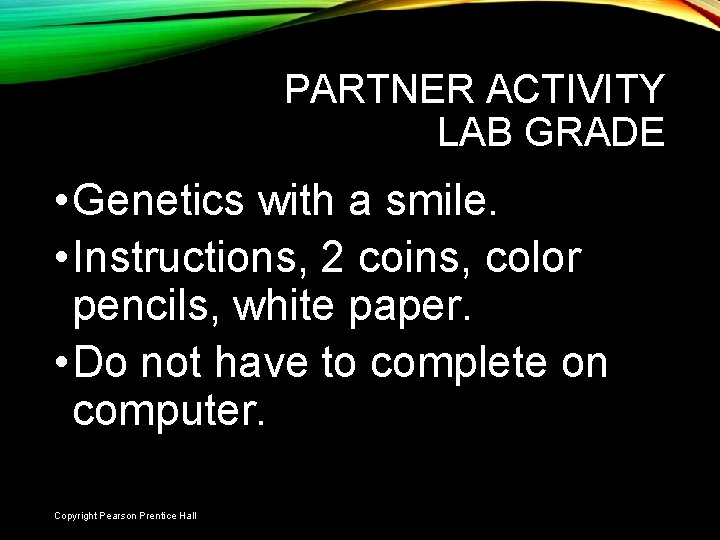PARTNER ACTIVITY LAB GRADE • Genetics with a smile. • Instructions, 2 coins, color pencils, white paper. • Do not have to complete on computer. Slide 33 of 21 Copyright Pearson Prentice Hall